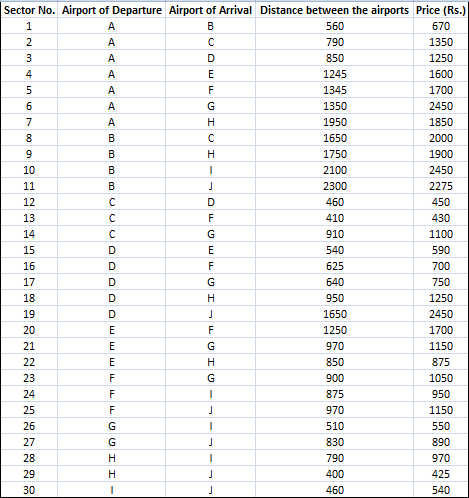### CAT 2007 Question 49

Instructions

DIRECTIONS for the following four questions:

A low-cost airline company connects ten India cities, A to J. The table below gives the distance between a pair of airports and the corresponding price charged by the company. Travel is permitted only from a departure airport to an arrival airport. The customers do not travel by a route where they have to stop at more than two intermediate airports.Question 49

# If the prices include a margin of 10% over the total cost that the company incurs, what is the minimum cost per kilometer that the company incurs in flying from A to J?

Solution

The minimum cost from A to J we know is 2275.

Let the CP to company be C

Since 10% over actual CP is the total price  i.e.  $$\text{CP}\times1.1 = 2275 \rightarrow CP = \frac{2275}{1.1}$$

The total distance is 1950+1400=2350 Km.

Cost per Km = $$\dfrac{\frac{2275}{1.1}}{2350}$$ = Rs 0.88/Km